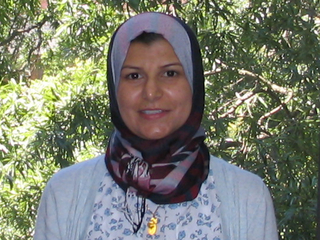# Doctoral Dissertation Defense: Sumaya Alzuhairy

## Advisors: Dr. Andrei Draganescu and Dr. Bedrich Sousedik

Friday, November 12, 2021
12:00 PM – 2:00 PMTitle:  Multilevel Methods For Optimal Control Of Elliptic Partial Differential Equation With Stochastic Coefficients

Abstract
The primary goal of the dissertation is to develop and analyze efficient algorithms for solving optimal control problems constrained by partial differential equations (PDEs) with stochastic coefficients (SPDECO). Over the last two decades significant progress has been achieved on developing efficient solvers for optimal control problems constrained by deterministic PDEs (PDECO). However, the challenges associated with PDECO, in particular their potentially large size due to discretization, pale in comparison to the exponentially growing size of SPDECO problems, where the curse of dimensionality associated with modeling stochastic components plays a decisive role.

This work focuses on a classical SPDECO model problem: a quadratic optimization function of tracking type constrained by a linear elliptic equation with a stochastic diffusion coefficient. As is customary in the computational mathematics community, the stochastic coefficient is expressed using a (truncated) generalized polynomial chaos expansion.  Furthermore, using the first-discretize-then-optimize approach, the stochastic elliptic PDE is discretized using a finite element framework for the spatial component. Finally, the SPDE is eliminated from the discrete SPDECO problem,  resulting in a potentially very large quadratic optimization problem, whose optimality condition is, naturally, a linear system. The preconditioning techniques in this dissertation apply to this linear system.

Two standard discretization methods are considered for the stochastic components: stochastic collocation (SC) using sparse grids, and the stochastic Galerkin method (SG). While both approaches lead to very high dimensional systems, the SC method has the advantage of being non-intrusive and only requiring independent deterministic PDE-solves, and so is highly parallelizable, and existing codes can be reused. On the other hand, the SG approach leads to a single linear system to be solved, but comes with the nontrivial added cost of an intricate reformulation of the problem. Each of the two approaches introduces a new complexity into the problem in addition to the spatial mesh size: for SC the problem size increases exponentially with the number for sparse grids levels, while for SG it is the polynomial degree that plays the same role.

In this dissertation we develop and analyze multilevel preconditioning techniques for both the SC- and SG-based discretizations of the SPDECO.  We show that, under certain assumptions on the SPDE, the preconditioner inherits the second-order optimality with respect to the spatial discretization parameter (the grid size). However, it is remarkable that the preconditioner exhibits robustness also with respect to the number of sparse grid levels (for SC) as well as polynomial degree (SG). Even more so, numerical computations show that robustness is persistent also with respect to the stochastic dimension, another parameter that radically influences the total size of the problem.
Tags: phd-defense